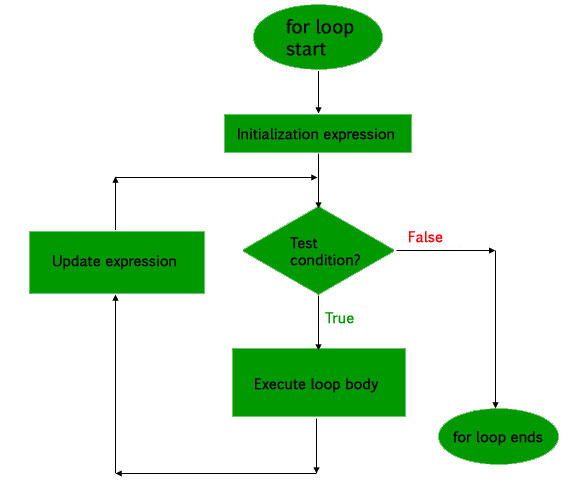Open in App
Not now

# PHP for Loop

• Last Updated : 25 Aug, 2022

The for loop is the most complex loop in PHP that is used when the user knows how many times the block needs to be executed. The for loop contains the initialization expression, test condition, and update expression (expression for increment or decrement).

Flowchart of for Loop:Syntax:

```for (initialization expression; test condition; update expression) {
// Code to be executed
}```

Loop Parameters:

• Initialization Expression: In this expression, we have to initialize the loop counter to some value. For example: \$num = 1;
• Test Condition: In this expression, we have to test the condition. If the condition evaluates to “true” then it will execute the body of the loop and go to the update expression otherwise it will exit from the for loop. For example: \$num <= 10;
• Update Expression: After executing the loop body, this expression increments/decrements the loop variable by some value. For example: \$num += 2;

Example 1: The following code shows a simple example using for loop.

## PHP

 ``

Output

```0
5
10
15```

Example 2: The following code shows another example of for loop.

## PHP

 ``

Output

```5
10
15
20
25
30
35
40
45```

My Personal Notes arrow_drop_up
Related Articles